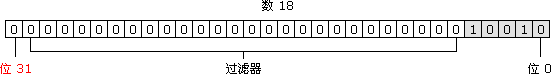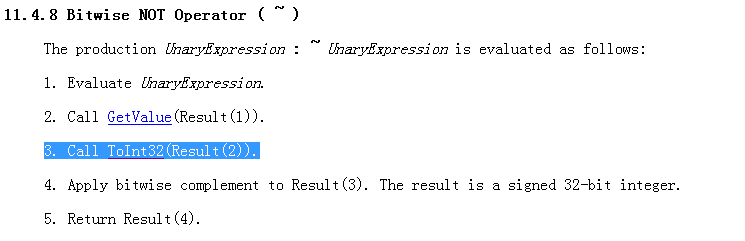# JavaScript - 如果...没有方法

JavaScript - 前端开发交流群：377786580

## 如果...没有方法

`Math.round()` 就是传说中的四舍五入啦...

``````Math.round(12.1);//12
Math.round(12.8);//13
Math.round(-12.1);//-12
Math.round(-12.8);//-13``````

## 开拓思路

``````//num===6.2
function round(num) {
var nums = String(num).split("."),//[6,2]
num0 = nums,//6
num1 = nums;//2

if (parseInt(num1.substring(0, 1)) < 5) { //2<5
return parseInt(num0);
} else {
if (num0 > 0) {
return parseInt(num0) + 1;
} else {//负数
return parseInt(num0) - 1;
}
}
}``````

``````//num===6.2
function round(num) {
var round_x = (((10 * num) % 10) > 0) ?
((10 * num) % 10) : //正数
-((10 * num) % 10);//负数

if (round_x < 5) {
return num - (num % 1);//把小数点后的的数字干掉
} else {
return (num > 0 ?
(num - (num % 1) + 1) : //正数
num - (num % 1) - 1); //负数
}
}``````

``````function round(num) {
var value = num > 0 ?
num + 0.5 :     //正数
-(num - 0.5);   //负数
value = value - value % 1;//得到新数的整数部分
//如果相差<1
return value - num < 1 ?
num - num % 1 :
value;
}``````

## 脑洞大开

``````//@Gray Zhang的"给跪版"，不支持负数
function round(x) {
return ~~(x + 0.5);
}

//@Gray Zhang的"给跪加深版"，支持正负数
function round(x) {
return ~~(x > 0 ? (x + 0.5) : (x - 0.5));
}

//@强子~Developer的"请收下我的膝盖版"
function round(x) {
return (x > 0 ? x + 0.5 : x - 0.5) | 0;
}``````

``````function round(x) {
return ~~(x + 0.5 + (x >> 30));
}``````

## 简单的分析

### 重温整数

ECMAScript 整数有两种类型，即有符号整数（允许用正数和负数）和无符号整数（只允许用正数）。在 ECMAScript 中，所有整数字面量默认都是有符号整数。 有符号整数使用 31 位表示整数的数值，用第 32 位表示整数的符号，0 表示正数，1 表示负数。数值范围从 -2147483648 到 2147483647。见下图：js中 `toString()` 方法可以 to 出二进制，而 `parsetInt()` 方法的第二个参数可以指定转换进制：

``````(18).toString(2) //"10010"
parseInt(10010,2) //18``````

### ~的运算过程

`~` 就是按位取反，类似： `00111` ，取反则为 `11000` 。 取反会干掉小数，`~` 运算符的运算过程可以戳 这里，我们看到调用了 `ToInt32()```````~~18.5          //18 - 等同于parseInt(18)
parseInt(18.5)  //18``````

`~~` 是按位取反再取反，本质上就是一个干掉小数的过程。

### >>有符号右移运算符

`>>` 是有符号右移运算符由两个大于号表示（>>）。它把 32 位数字中的所有数位整体右移，同时保留该数的符号（正号或负号）。有符号右移运算符恰好与左移运算相反。

``-2>>30 // -1 （感谢群里的@Superior和@Jeff Xiao提供）``

• 1 0000000000000000000000000000010 //-2二进制
• 1 1111111111111111111111111111110 //-2进行补码
• 1 1111111111111111111111111111111 //向右移动30，高位以符号位（第32位）补全
• 1 0000000000000000000000000000001 //因为符号位为符号，所以是负数，则补码形式存储，还原为-1

``~~(x + 0.5 + (x >> 30))``
• 假设X是-12.5
• 首先，-12.5+0.5===-12
• -12.5>>30：上面我们说过，ECMAScript有符号整数使用`31`位表示整数的数值，所以在ECMAScript中，任何一个数右移30位得到的结果只能是2种：正数得到0，负数得到-1。
• -12-1===-13

JavaScript - 前端开发交流群：377786580

2223